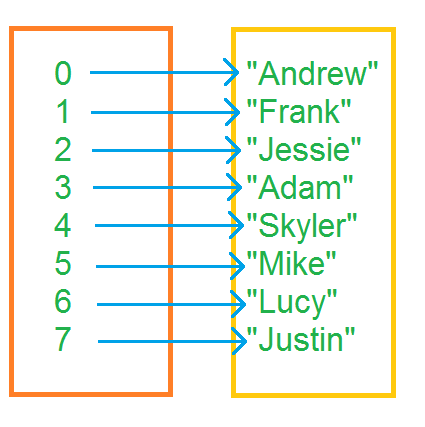# Part 3.3Arrays in PHP

## What is an array?

Put simply, an array is a collection of other items. In programming, an array can be seen to similar to the way that variables are stored in memory. In this sense, there is a direct mapping between the variable name or index and the value.

Arrays are incredibly useful ways of storing lots of information in one easy to access place. Not only this, unlike 1-dimension variables (all variables used in this tutorial), array values can be defined at runtime.

As mentioned, arrays can be seen as maps:An array provides a map from the left (index or key) to something on the right (value)

## Arrays in PHP

### Declaring arrays

In PHP, unlike certain other languages, arrays are dynamically sized meaning that the size can change at any point without having to redeclare the array (this may go on in the background in the PHP interpreter but the language itself does not require this).

An array in PHP is declared using the array keyword and displayed using print_r function:

PHP
<?php
$array = array(); print_r($array);
?>


This defines an empty array that is, one with no elements within it. The array need not be empty on the instantiation of the array:

PHP
<?php
$array = array("Firstname", "Surname"); print_r($array);
?>


PHP 5.4 also defines a new syntax that is similar to most other languages that does not require the use of the array construct:

PHP
<?php
$array = ["Firstname", "Surname"]; print_r($array);
?>

Take note that although a new array is defined using the array() syntax which resembles a function call, it is in fact not a function call at all and the array token is actually a keyword in the PHP specification.

### Adding elements to an array

PHP the use of the array_push function to put an element in the array:

PHP
<?php
$array = array(); array_push($array, "Value");
print_r($array); ?>  Arrays can also be given a value at a certain index using: PHP <?php$array = array("Firstname", "Surname");
$array = "Jack";$array = "Spillburg";
print_r($array); ?>  Arrays can also be given mixed values in PHP, so an array that has a collection of string values can have integers added to it and vice versa. ### Retrieving elements from an array Like many other languages, PHP defines a simple way of getting a value from an array. PHP uses the index (a numeric location in the array) or a key to retrieve a value from the array. Using the index, the keys start at the number 0 and count up so the first index is 0. PHP <?php$array = array();
$array = "Jack";$array = "Spillburg";
print_r($array); ?>  ### Retrieving elements from an associative array Elements can be retrieved the same way with associative arrays as with standard arrays except that instead of the index, the key is given: PHP <?php$array = array("Jack" => "Adams");
echo \$array["Jack"];
?>

Feedback 👍
Comments are sent via email to me.